Updating search results...

# 9 Results

View
Selected filters:
• UT.MATH.III.A.SSE.1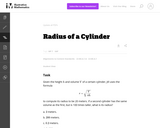Unrestricted Use
CC BY
Rating
0.0 stars

This is a task from the Illustrative Mathematics website that is one part of a complete illustration of the standard to which it is aligned. Each task has at least one solution and some commentary that addresses important asects of the task and its potential use. Here are the first few lines of the commentary for this task: Given the height $h$ and volume $V$ of a certain cylinder, Jill uses the formula r=\sqrt{\frac{V}{\pi h}} to compute its radius to be 20 meters. If a s...

Subject:
Mathematics
Secondary Mathematics
Material Type:
Activity/Lab
Provider:
Illustrative Mathematics
Provider Set:
Illustrative Mathematics
Author:
Illustrative Mathematics
03/20/2013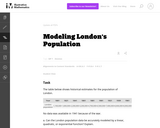Unrestricted Use
CC BY
Rating
0.0 stars

This is a task from the Illustrative Mathematics website that is one part of a complete illustration of the standard to which it is aligned. Each task has at least one solution and some commentary that addresses important asects of the task and its potential use. Here are the first few lines of the commentary for this task: The table below shows historical estimates for the population of London. Year18011821 18411861 18811901 1921 1939 1961 London population 1,100,000 1,60...

Subject:
Mathematics
Secondary Mathematics
Material Type:
Activity/Lab
Provider:
Illustrative Mathematics
Provider Set:
Illustrative Mathematics
Author:
Illustrative Mathematics
10/30/2013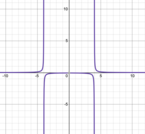Conditional Remix & Share Permitted
CC BY-NC
Rating
0.0 stars

This lesson includes a video&nbsp;lesson I did with my Secondary 3 Honors class that is a pre-lesson to graphing rational functions. In this video, we identify the horizontal and vertical asymptotes, holes, domain and range, and the x and y intercepts of rational functions.&nbsp; There is guided notes, a homework assignment, and a bell quiz also attached.

Subject:
Secondary Mathematics
Material Type:
Lesson
Author:
Tyrani Bevell
10/27/2021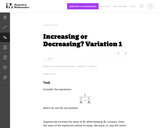Unrestricted Use
CC BY
Rating
0.0 stars

Students are asked to consider the expression that arises in physics as the combined resistance of two resistors in parallel. However, the context is not explicitly considered here. The task is good general preparation for problems more specifically aligned to either A-SSE.1 or A-SSE.2.

Subject:
Mathematics
Secondary Mathematics
Material Type:
Activity/Lab
Provider:
Illustrative Mathematics
Provider Set:
Illustrative Mathematics
Author:
Illustrative Mathematics
05/01/2012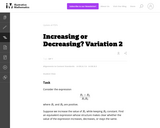Unrestricted Use
CC BY
Rating
0.0 stars

The purpose of this task is to help students see manipulation of expressions as an activity undertaken for a purpose.

Subject:
Mathematics
Secondary Mathematics
Material Type:
Activity/Lab
Provider:
Illustrative Mathematics
Provider Set:
Illustrative Mathematics
Author:
Illustrative Mathematics
05/01/2012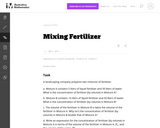Unrestricted Use
CC BY
Rating
0.0 stars

The problem deals with a rational expression which is built up from operations arising naturally in a context: adding the volumes of the fertilizer and the water, and dividing the volume of the fertilizer by the resulting sum. Thus it encourages students to see the expression as having meaning in terms of numbers and operations, rather than as an abstract arrangement of symbols.

Subject:
Mathematics
Secondary Mathematics
Material Type:
Activity/Lab
Provider:
Illustrative Mathematics
Provider Set:
Illustrative Mathematics
Author:
Illustrative Mathematics
05/01/2012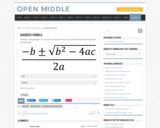Restricted Use
Rating
0.0 stars

Open Middle provides math problems that have a closed beginning, a closed end, and an open middle. This means that there are multiple ways to approach and ultimately solve the problems. Open middle problems generally require a higher Depth of Knowledge than most problems that assess procedural and conceptual understanding.

Subject:
Mathematics
Material Type:
Activity/Lab
Provider:
Open Middle Math
04/26/2022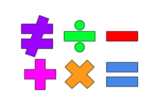Unrestricted Use
Public Domain
Rating
0.0 stars

This lesson is for a math classroom, but can be adapted to fit any grade, subject, or content.&nbsp;&nbsp;In this lesson, students will use an iPad and its features: Keynote, Pages, Garageband, Numbers, Presentation, and iMovie.&nbsp; Students will use 3 of those features/programs to create a video lesson consisting of several examples from a topic of their choice.&nbsp; This project is in place of a term final, so their chosen topic should be from their current term.Image citation: The image is one I created.&nbsp;&nbsp;

Subject:
Secondary Mathematics
Material Type:
Assessment
Author:
Tyrani Bevell
02/26/2022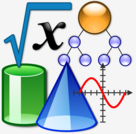Conditional Remix & Share Permitted
CC BY-NC
Rating
0.0 stars

Thumbnail photo:&nbsp;&nbsp;&quot;File:Nuvola Math and Inf.svg&quot;&nbsp;by&nbsp;Vojtěch Pikal&nbsp;is marked with&nbsp;CC0 1.0&nbsp;&nbsp;This is a lesson plan that can be used in any class and for any subject.&nbsp; The idea is to have the students review the content learned over the term, pick one of the concepts, and create a mini video lesson on the chosen concept.&nbsp; This is a project in place of a term final, but can be used at any time!I have tagged only a few math standards because this lesson applies to all content and all standards.

Subject:
Other
Material Type:
Lesson
Author:
Tyrani Bevell